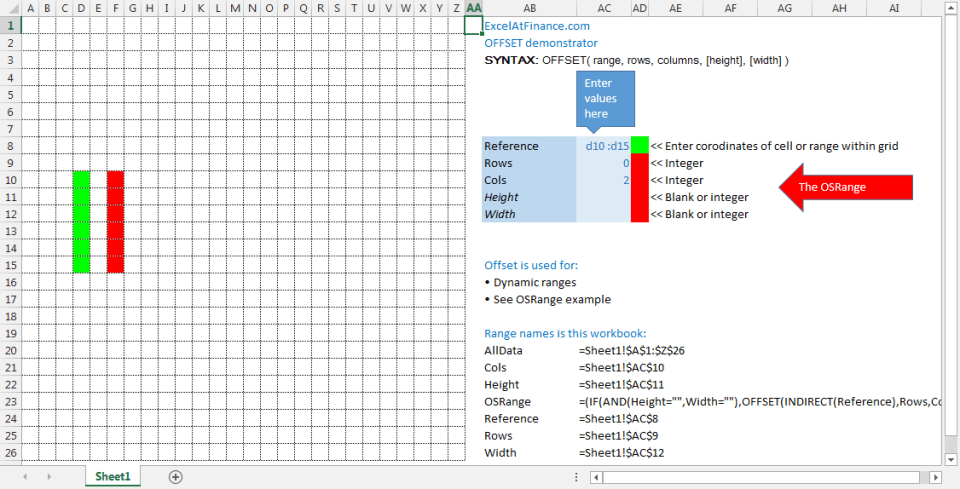# Offset function

## Dynamic ranges

The module demonstrates the Excel OFFSET function in the context of dynamic ranges. The module is designed for use by participants of the xlf presentation series.

The stock analyser project: In figure 1, the Reference (green) is similar to the DateVector primary key of dates in the Stock Analyser Project, and the red vector has the properties of a DataVector. The range shown by the "Enter values here" label serves the same function as a Selector Panel.

The important part of this demonstration is the dynamic offset range named OSRange. Download the xlsm file and change some of the selector values to see the changes in the position and dimensions of the OSRange. Because the Height and Width values are optional, they can be left blank. If so, then the OSRange will adopt the proportions of the Reference. In the Stock Analyser Project, the important argument is the cols value. The DataVector will generally be in the same row range as the DataVector.

In figure 1, if the OSRange is over the edge of the worksheet, OFFSET returns a #REF! error value.Fig 1: The OFFSET dynamic range demonstrator - The OFFSET reference is shown in green, and the dynamic vector, named OSRange is shown in red. The user can manipulate both ranges by changing the values in the "Enter values here" range (AC8:AC12).

For advanced Excel users, the next section discusses the construction of the OSRange name. It consists of a series of nested IF statements. This specific material is not required in the Stock Analyser Project.

## Writing the OSRange formula

The formula is based on the INDIRECT function (returns the reference specified by a text string) and the OFFSET function (returns a reference to a range that is a specified number of rows and columns from a cell or range of cells).

• SYNTAX: INDIRECT(ref_text,a1)
• SYNTAX: OFFSET(reference, rows, cols, [height], [width])

The OSRange is written as:

• OSRange: =(IF(AND(Height="",Width=""),OFFSET(INDIRECT(Reference),Rows,Cols),IF(Width="",OFFSET(INDIRECT(Reference),Rows,Cols,Height),IF(Height="",OFFSET(INDIRECT(Reference),Rows,Cols,,Width),OFFSET(INDIRECT(Reference),Rows,Cols,Height,Width)))))

### Discussion

The OFFSET function has 2 optional arguments: height, and width. In the figure 1 example, these values come from the range names Height and Width. A problem occurs if either of these values is omitted. If the cell Width is left blank, then the width argument takes the value of zero. The same applies of the Height value. In the case were Height and/or Width are omitted, the OSRange should take on the dimension of Reference, but instead it is Height: zero, and Width: zero, thus - nothing is returned.

To solve this problem, an OFFSET function is written for each of the four cases. Each of the 4 cases is included in a nested IF structure. This is best shown by dismantling the OSRange name.

1. Omit both Height and Width arguments: IF(AND(Height="",Width=""),OFFSET(INDIRECT(Reference),Rows,Cols)
2. Omit the Width argument: IF(Width="",OFFSET(INDIRECT(Reference),Rows,Cols,Height)
3. Omit the Height argument: IF(Height="",OFFSET(INDIRECT(Reference),Rows,Cols,,Width)
4. Include both optional arguments - the default case: OFFSET(INDIRECT(Reference),Rows,Cols,Height,Width)